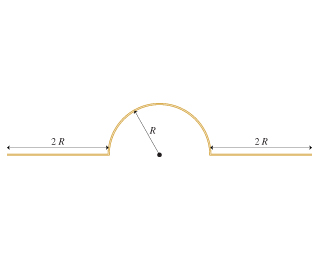# Problem: What is the electric potential at the center of the semicircle?Give your answer in terms of λ, R, ϵ 0 and appropriate constant

###### FREE Expert Solution

The electric potential:

$\overline{){\mathbf{V}}{\mathbf{=}}\frac{\mathbf{k}\mathbf{q}}{\mathbf{r}}}$

The charge carried by the piece of wire is given by,

$\overline{){\mathbf{d}}{\mathbf{q}}{\mathbf{=}}{\mathbf{\lambda }}{\mathbf{d}}{\mathbf{x}}}$, where λ is the linear charge density.

To calculate the electric potential at the center of the semicircle due to the right wire:

dVright = kdq/x

Integrating both sides from x = R to 3R:

$\begin{array}{rcl}{\mathbf{V}}_{\mathbf{r}\mathbf{i}\mathbf{g}\mathbf{h}\mathbf{t}}& \mathbf{=}& {\mathbf{\int }}_{\mathbf{R}}^{\mathbf{3}\mathbf{R}}\mathbf{\left(}\frac{\mathbf{k}\mathbf{d}\mathbf{q}}{\mathbf{x}}\mathbf{\right)}\\ & \mathbf{=}& {\mathbf{\int }}_{\mathbf{R}}^{\mathbf{3}\mathbf{R}}\mathbf{\left(}\frac{\mathbf{k}\mathbf{\lambda }\mathbf{d}\mathbf{x}}{\mathbf{x}}\mathbf{\right)}\\ & \mathbf{=}& \mathbf{k}\mathbf{\lambda }{\mathbf{\left[}\mathbf{l}\mathbf{n}\mathbf{x}\mathbf{\right]}}_{\mathbf{R}}^{\mathbf{3}\mathbf{R}}\end{array}$

91% (195 ratings)###### Problem Details

What is the electric potential at the center of the semicircle?

Give your answer in terms of λ, R, ϵ 0 and appropriate constant